## 20 Questions MCQ Test Maths Olympiad Class 6 | Olympiad Test : Decimals

Description
Attempt Olympiad Test : Decimals | 20 questions in 40 minutes | Mock test for Class 6 preparation | Free important questions MCQ to study Maths Olympiad Class 6 for Class 6 Exam | Download free PDF with solutions
QUESTION: 1

Solution:
QUESTION: 2

### 0.4 + 0.004 + 4.4 = ?

Solution:

Here,
? = 0.4 + 0.004 + 4.4 = 4.804

QUESTION: 3

### 1.103 – 0.3 = ?

Solution:

? = 1.103 – 0.3 = 0.803

QUESTION: 4

3 – 0.007 = ?

Solution:

? = 3 – 0.007 = 2.993

QUESTION: 5

Among 7.6, 7.006, 7.66 and 7.08, which is the largest number?

Solution:

∴ 7.006 < 7.08 < 7.6 < 7.66
∴ 7.66 is the largest number

QUESTION: 6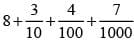Solution:

Given expression can be written as
8 + 0.3 + 0.04 + 0.007 = 8.347

QUESTION: 7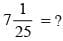Solution: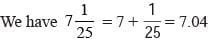QUESTION: 8

6 – 0.23 + 1.2 – 5.76 = ?

Solution:

? = 6 – 0.23 + 1.2 – 5.76
= 7.2 – 5.99 = 1.21

QUESTION: 9

37 – 35.79 = ?

Solution:

? = 37 – 35.79 = 1.21

QUESTION: 10

What is to be added to 74.5 to get 81?

Solution:

Required number = 81 – 74.5 = 6.5

QUESTION: 11

What is to be subtracted from 7.3 to get 0.867?

Solution:

Required number = 7.3 – 0.867 = 6.433

QUESTION: 12

By how much should 79.5 be decreased to get 27.89 ?

Solution:

Required number = 79.5 – 27.89 = 51.61

QUESTION: 13

By how much should 32.754 by increased to get 53?

Solution:

Required number = 53 – 32.754 = 20.246

QUESTION: 14

76.3 – 7.666 – 6.77 – 5.55 = ?

Solution:

? = 76.3 – 7.666 – 6.77 – 5.55
= 76.3 – 19.986 = 56.314

QUESTION: 15

37 mm = ?

Solution: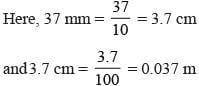QUESTION: 16

2 kg 57 g = ?

Solution:

2 kg 57 g = 2 × 1000 + 57 = 2057 g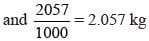QUESTION: 17

During three days of a week, an auto driver earns Rs 302.80, Rs 379.20 and Rs 297.60 respectively. What is his total earning during these days?

Solution:

Total earning = 302.80 + 379.20 + 297.60 = 979.60

QUESTION: 18

What is the decimal equivalent of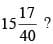Solution: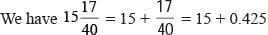= 15.425

QUESTION: 19

Mohan purchased 5 kg 75 g of fruits and 3kg 465g of vegetables and put them in a bag. If this bag with these contents weights 9 kg. What is the weight of empty bag?

Solution:

Here, 5kg 75g + 3kg 465g = 8kg 540g
∴ 9kg - 8kg 540g = 460g

QUESTION: 20

What should be subtracted from the sum of 4.902 & 15.376 to get 16.307 ?

Solution:

Here, 4.902 + 15.376 = 20.278
then 20.278 – 16.307 = 3.971Use Code STAYHOME200 and get INR 200 additional OFF Use Coupon Code